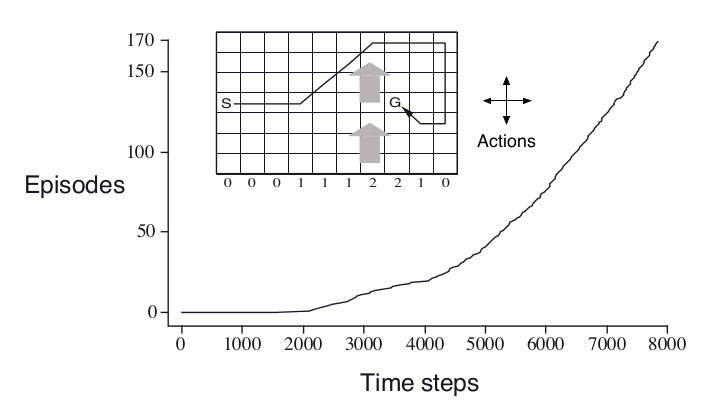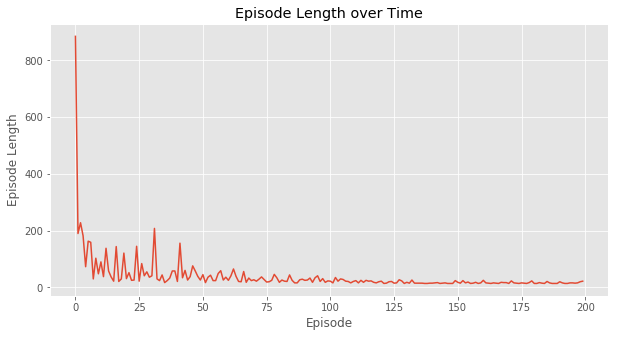# 强化学习简介(四)

Posted by lili on

## 时间差分预测(TD Prediction)

$V(S_t) \leftarrow V(S_t)+\alpha [G_t-V(S_t)]$

$V(S_t) \leftarrow V(S_t)+\alpha [R_{t+1}+\gamma V(S_{t+1})-V(S_t)]$

$\begin{split} v_\pi(s) & \equiv \mathbb{E}_\pi[G_t|S_t=s] \\ & =\mathbb{E}_\pi[R_{t+1}+\gamma G_{t+1}|S_t=s] \\ & =\mathbb{E}_\pi[R_{t+1}+\gamma v_\pi{S_{t+1}}|S_t=s] \end{split}$

$\delta_t \equiv R_{t+1}+\gamma V(S_{t+1}) -V(S_t)$

$\begin{split} G_t-V(S_t) & =R_{t+1}+\gamma G_{t+1}-V(S_t) +\gamma V(S_{t+1}) -\gamma V(S_{t+1}) \\ & =\delta_t+\gamma(G_{t+1}-V(S_{t+1}))\\ & =\delta_t+\gamma \delta_{t+1}+\gamma^2(G_{t+2}-V(S_{t+2}))\\ & =\delta_t+\gamma \delta_{t+1}+\gamma^2 \delta_{t+2} + ... +\gamma^{T-t-1}\delta_{T-1}+\gamma^{T-t}(G_T-V(S_t))\\ & =\delta_t+\gamma \delta_{t+1}+\gamma^2 \delta_{t+2} + ... +\gamma^{T-t-1}\delta_{T-1}+\gamma^{T-t}(0-0))\\ & =\sum_{k=t}^{T-1}\gamma^{k-t}\delta_k \end{split}$

## Driving Home例子图：Driving Home示例

## TD和MC的比较

TD相对于MC最大的优点当然就是它是online的算法，不用等到Episode结束就可以更新，因此也就可以用于连续的任务。此外，TD通常收敛的更快，当然这只是经验，并没有理论的证明。$G_t=R_{t+1}+\gamma R_{t+2}+…+\gamma^{T-1}R_T$是$v_\pi(S_t)$的无偏估计，而”真实“的TD更新目标$R_{t+1}+\gamma v_\pi(S_{t+1})$也是无偏估计，但是因为我们并不知道$v_\pi(S_{t+1})$而是用$V(S_{t+1})$来近似的，也就是我们实际用的TD更新目标是$R_{t+1}+\gamma V(S_{t+1})$。这个目标是有偏的估计。因为MC依赖很多随机的Action、随机的状态跳转和随机的reward，所以它的估计方差较大，而TD值有一次随机的Action、随机的状态跳转和随机的reward，因此方差较小。此外MC因为有bootstrapping，因此它的收敛也依赖于初始值。

A,0,B,0
B,1 6次
B,0


(A,0,B,0)表示初始化状态A，进入B,reward是0，然后进入B，reward是0，结束。

$L=\sum_{k=1}^K\sum_t^{T_k}(G_t^k-V(S_t^k)^2)$

$\begin{split} \hat{P}_{s,s'}^a & =\frac{}{N(s,a)}\sum_{k=1}^K\sum_{t=1}^{T_k}1(s_t^k,a_t^k,s_{t+1}^k=s,a,s') \\ \hat{R}_s^a & =\frac{1}{N(s,a)}\sum_{k=1}^K\sum_{t=1}^{T_k}1(s_t^k,a_t^k=s,a)R_t^k \end{split}$

## SARSA

$Q(S_t,A_t) \leftarrow Q(S_t,A_t)+\alpha [R_{t+1}+\gamma Q(S_{t+1},A_{t+1})-Q(S_t)]$

## Windy Gridworld环境介绍图：Windy Gridworld

super(WindyGridworldEnv, self).__init__(nS, nA, P, isd)


P是一个dict，key是(0-69)，表示每个状态的转移概率。注意：我们用二维数组表示状态，但是DiscreteEnv要求状态是一维的。我们需要在二维和一维之间进行转换，这里会用到numpy.ravel_multi_index函数。我们通过几个例子来学习这个函数：

        >>> arr = np.array([[3,6,6],[4,5,1]])
>>> np.ravel_multi_index(arr, (7,6))
array([22, 41, 37])


	>>> arr = np.array([[3,6,6],[4,5,1]])
>>> np.ravel_multi_index(arr, (7,6), order='F')
array([31, 41, 13])


(3,4)表示第3行第4列，因为是列优先，所以4*7+3=31。

# C风格的顺序
0 1 2
3 4 5
# Fortrain风格的顺序
0 2 4
1 3 5


{
0: [(1.0, 6, -1.0, False)],
1: [(1.0, 7, -1.0, False)],
2: [(1.0, 6, -1.0, False)],
3: [(1.0, 5, -1.0, False)]
}


{
0: [(1.0, 0, -1.0, False)],
1: [(1.0, 1, -1.0, False)],
2: [(1.0, 10, -1.0, False)],
3: [(1.0, 0, -1.0, False)]
}


(0, 0)是最左上的点，它往上(0)和往左(3)都越界(碰墙)，因此还是呆在原地不到。

{
0: [(1.0, 28, -1.0, False)],
1: [(1.0, 39, -1.0, False)],
2: [(1.0, 48, -1.0, False)],
3: [(1.0, 37, -1.0, True)]
}


isd(Initial State Distribution)表示初始状态的分布，这是一个长度为70的数组，表示初始处于这个状态的概率，我们这里返回的isd只有在下标30(对应的二维下标是(3,0))是1，其余都是零，也就是初始状态总是在第四行第一列。

UP = 0
RIGHT = 1
DOWN = 2
LEFT = 3

class WindyGridworldEnv(discrete.DiscreteEnv):

# 把范围限定为(0, shape-1)和(0, shape-1)内。
def _limit_coordinates(self, coord):
coord = min(coord, self.shape - 1)
coord = max(coord, 0)
coord = min(coord, self.shape - 1)
coord = max(coord, 0)
return coord

# 计算P(s'|s,a)
def _calculate_transition_prob(self, current, delta, winds):
# current是2-tuple，分别表示横纵轴坐标
# delta是移动方向，比如向右异地是(0,1)，向上是(-1,0)
# 计算方法是把current和delta的对应坐标相加，同时在加上往上(-1,0)的风乘以风力。
# 风力存在winds数组里，winds[1,2]表示下标为(1,2)点的风力
new_position = np.array(current) + np.array(delta) + np.array([-1, 0]) * winds[tuple(current)]
new_position = self._limit_coordinates(new_position).astype(int)
new_state = np.ravel_multi_index(tuple(new_position), self.shape)
is_done = tuple(new_position) == (3, 7)
return [(1.0, new_state, -1.0, is_done)]

def __init__(self):
self.shape = (7, 10)

nS = np.prod(self.shape)
nA = 4

# 风力
winds = np.zeros(self.shape)
# 第4,5,6,9列的风力为1
winds[:,[3,4,5,8]] = 1
# 第7和8列的风力为2
winds[:,[6,7]] = 2

# 计算P(s'|s,a)
P = {}
for s in range(nS):
position = np.unravel_index(s, self.shape)
P[s] = { a : [] for a in range(nA) }
P[s][UP] = self._calculate_transition_prob(position, [-1, 0], winds)
P[s][RIGHT] = self._calculate_transition_prob(position, [0, 1], winds)
P[s][DOWN] = self._calculate_transition_prob(position, [1, 0], winds)
P[s][LEFT] = self._calculate_transition_prob(position, [0, -1], winds)

# 初始状态在 (3, 0)
isd = np.zeros(nS)
isd[np.ravel_multi_index((3,0), self.shape)] = 1.0

super(WindyGridworldEnv, self).__init__(nS, nA, P, isd)

def render(self, mode='human', close=False):
if close:
return

outfile = StringIO() if mode == 'ansi' else sys.stdout

for s in range(self.nS):
position = np.unravel_index(s, self.shape)
# print(self.s)
if self.s == s:
output = " x "
elif position == (3,7):
output = " T "
else:
output = " o "

if position == 0:
output = output.lstrip()
if position == self.shape - 1:
output = output.rstrip()
output += "\n"

outfile.write(output)
outfile.write("\n")


env = WindyGridworldEnv()

print(env.reset())
env.render()

print(env.step(1))
env.render()

print(env.step(1))
env.render()

print(env.step(1))
env.render()

print(env.step(1))
env.render()


30
o  o  o  o  o  o  o  o  o  o
o  o  o  o  o  o  o  o  o  o
o  o  o  o  o  o  o  o  o  o
x  o  o  o  o  o  o  T  o  o
o  o  o  o  o  o  o  o  o  o
o  o  o  o  o  o  o  o  o  o
o  o  o  o  o  o  o  o  o  o

(31, -1.0, False, {'prob': 1.0})
o  o  o  o  o  o  o  o  o  o
o  o  o  o  o  o  o  o  o  o
o  o  o  o  o  o  o  o  o  o
o  x  o  o  o  o  o  T  o  o
o  o  o  o  o  o  o  o  o  o
o  o  o  o  o  o  o  o  o  o
o  o  o  o  o  o  o  o  o  o

(32, -1.0, False, {'prob': 1.0})
o  o  o  o  o  o  o  o  o  o
o  o  o  o  o  o  o  o  o  o
o  o  o  o  o  o  o  o  o  o
o  o  x  o  o  o  o  T  o  o
o  o  o  o  o  o  o  o  o  o
o  o  o  o  o  o  o  o  o  o
o  o  o  o  o  o  o  o  o  o

(33, -1.0, False, {'prob': 1.0})
o  o  o  o  o  o  o  o  o  o
o  o  o  o  o  o  o  o  o  o
o  o  o  o  o  o  o  o  o  o
o  o  o  x  o  o  o  T  o  o
o  o  o  o  o  o  o  o  o  o
o  o  o  o  o  o  o  o  o  o
o  o  o  o  o  o  o  o  o  o

(24, -1.0, False, {'prob': 1.0})
o  o  o  o  o  o  o  o  o  o
o  o  o  o  o  o  o  o  o  o
o  o  o  o  x  o  o  o  o  o
o  o  o  o  o  o  o  T  o  o
o  o  o  o  o  o  o  o  o  o
o  o  o  o  o  o  o  o  o  o
o  o  o  o  o  o  o  o  o  o


## SARSA代码

def sarsa(env, num_episodes, discount_factor=1.0, alpha=0.5, epsilon=0.1):
"""
SARSA 算法: On-policy TD控制。找到最优的ε-greedy 策略。

参数:
env: OpenAI 环境。
num_episodes: 采样的episodes次数。
discount_factor: 打折因子。
alpha: TD学习率(learning rate)。
epsilon:  ε-贪婪的ε，随机行为的概率

返回值:
二元组(Q, stats).
Q 是最优行为价值函数，一个dictionary state -> action values.
stats是一个EpisodeStats对象，这个对象有两个numpy数值，分别是
episode_lengths和episode_rewards，存储每个Episode的长度和奖励，用于绘图。
"""

# 最终返回的Q(s,a)函数The final action-value function。
# 它是一个嵌套的dictionary state -> (action -> action-value)。
Q = defaultdict(lambda: np.zeros(env.action_space.n))

# 参考返回值的注释。
stats = plotting.EpisodeStats(
episode_lengths=np.zeros(num_episodes),
episode_rewards=np.zeros(num_episodes))

# 通过Q(s,a)得到ε-贪婪的策略，注意Q变化后策略就随着改变了。
policy = make_epsilon_greedy_policy(Q, epsilon, env.action_space.n)

for i_episode in range(num_episodes):
if (i_episode + 1) % 100 == 0:
print("\rEpisode {}/{}.".format(i_episode + 1, num_episodes), end="")
sys.stdout.flush()

# 重置环境并且采样第一个Action
state = env.reset()
action_probs = policy(state)
action = np.random.choice(np.arange(len(action_probs)), p=action_probs)

for t in itertools.count():
# 采取行为A
next_state, reward, done, _ = env.step(action)

# 选择下一个行为A'
next_action_probs = policy(next_state)
next_action = np.random.choice(np.arange(len(next_action_probs)), p=next_action_probs)

# 更新统计信息
stats.episode_rewards[i_episode] += reward
stats.episode_lengths[i_episode] = t

# TD Update
td_target = reward + discount_factor * Q[next_state][next_action]
td_delta = td_target - Q[state][action]
Q[state][action] += alpha * td_delta

if done:
break

action = next_action
state = next_state

return Q, stats


## Q-学习(Q-Learning)

$\rho_{t:T+1}=\prod_{k=t}^{T}\frac{\pi(A_k|S_k)}{b(A_k|S_k)}$

V(s)的更新公式是：

$V(S_t) \leftarrow V(S_t)+\alpha(\rho_{t:T+1}G_t-V(S_t))$

$V(S_t) \leftarrow V(S_t)+\alpha(\rho_{t:T+1}(R_{t+1}+\gamma V(S_{t+1}))-V(S_t))$

Q-Learning有两个策略，基于Q(s,a)的贪心策略，这是目标策略；基于Q(s,a)的ε-贪婪策略，这是行为策略。此外Q-Learning不使用重要性采样，因此$\rho$是1。因此Q-Learning的更新公式是：

$Q(S_t,A_t) \leftarrow Q(S_t,A_t)+\alpha(R_{t+1}+\gamma V(S_{t+1},A')-Q(S_t,A_t))$

$\pi(S_{t+1})=arg\max_{a'} Q(S_{t+1},a')$

$\begin{split} & R_{t+1}+\gamma V(S_{t+1},A')-Q(S_t,A_t) \\ & =R_{t+1}+\gamma V(S_{t+1},arg\max_{a'} Q(S_{t+1},a'))-Q(S_t,A_t) \\ & =R_{t+1}+\gamma \max_aV(S_{t+1},a)-Q(S_t,A_t) \end{split}$

## Q-Learning代码

def q_learning(env, num_episodes, discount_factor=1.0, alpha=0.5, epsilon=0.1):
"""
Q-Learning 算法: Off-policy TD控制，它会采样ε-贪婪的策略来生成行为，并且使用贪婪的策略找到最优策略

参数:
env: OpenAI 环境。
num_episodes: 采样的episode次数
discount_factor: 打折因子。
alpha: TD学习率
epsilon: ε

返回值:
二元组(Q, stats).
Q 是最优行为价值函数，一个dictionary state -> action values.
stats是一个EpisodeStats对象，这个对象有两个numpy数值，
分别是episode_lengths和episode_rewards，存储每个Episode的长度和奖励，用于绘图。
"""

# 最终返回的Q(s,a)函数The final action-value function。
# 它是一个嵌套的dictionary state -> (action -> action-value)。
Q = defaultdict(lambda: np.zeros(env.action_space.n))

# 参考返回值的注释。
stats = plotting.EpisodeStats(
episode_lengths=np.zeros(num_episodes),
episode_rewards=np.zeros(num_episodes))

# 通过Q(s,a)得到ε-贪婪的策略，注意Q变化后策略就随着改变了。
policy = make_epsilon_greedy_policy(Q, epsilon, env.action_space.n)

for i_episode in range(num_episodes):
if (i_episode + 1) % 100 == 0:
print("\rEpisode {}/{}.".format(i_episode + 1, num_episodes), end="")
sys.stdout.flush()

# 重置环境
state = env.reset()

for t in itertools.count():

# 使用ε-贪婪的策略采样一个Action
action_probs = policy(state)
action = np.random.choice(np.arange(len(action_probs)), p=action_probs)
next_state, reward, done, _ = env.step(action)

# 更新统计信息
stats.episode_rewards[i_episode] += reward
stats.episode_lengths[i_episode] = t

# TD Update
best_next_action = np.argmax(Q[next_state])
td_target = reward + discount_factor * Q[next_state][best_next_action]
td_delta = td_target - Q[state][action]
Q[state][action] += alpha * td_delta

if done:
break

state = next_state

return Q, stats图：Q-Learning学习过程